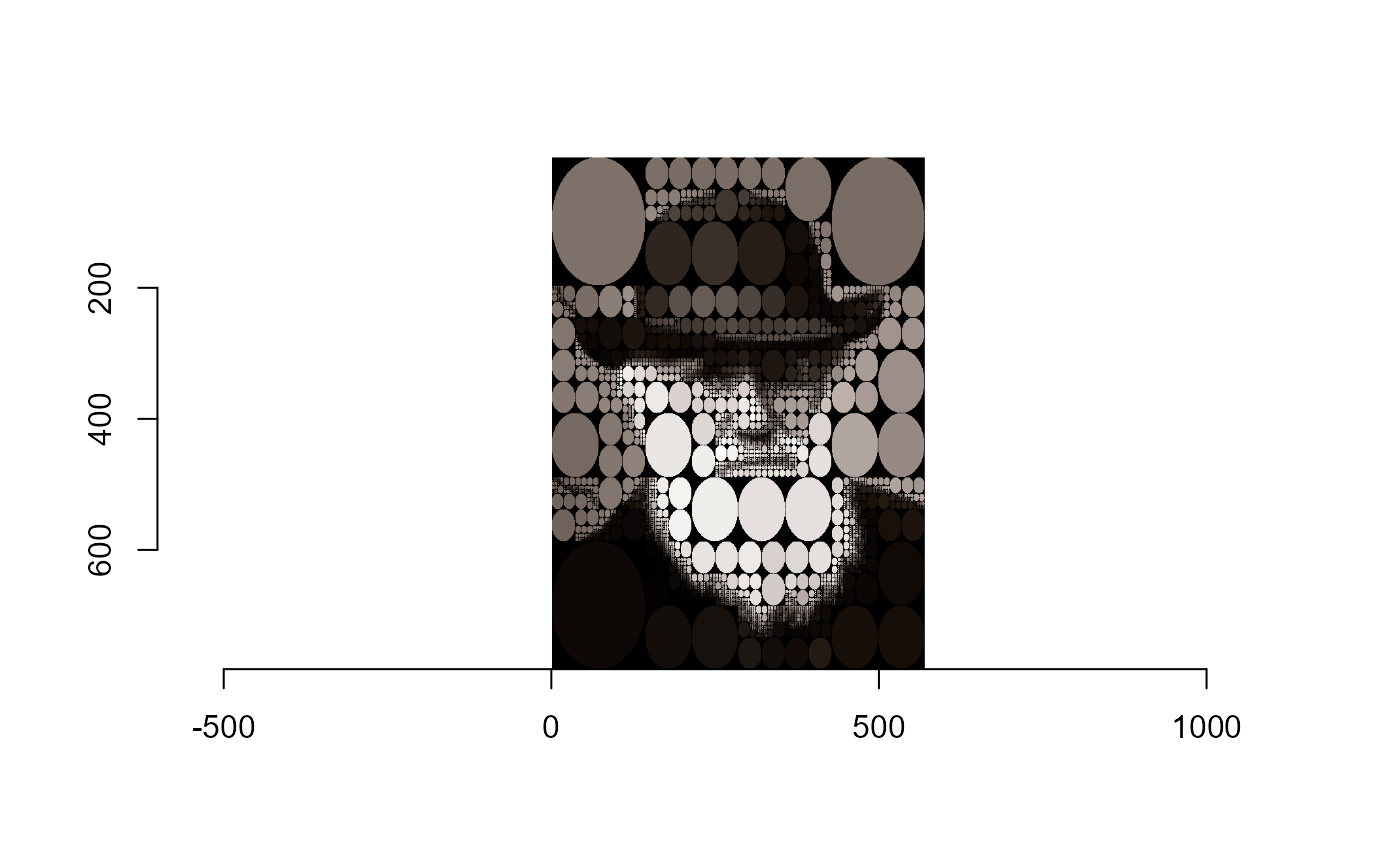This article goes over a few examples of using the trampoline package to do recursive tree traversals (without fear of hitting the limits of R’s call stack).

# Example 1: Phylogenetic Tree Clade Collapse

library(trampoline)
library(ape)
library(imager)
#> Warning: package 'imager' was built under R version 4.0.5
#> Warning: package 'magrittr' was built under R version 4.0.3
#>
#> Attaching package: 'imager'
#> The following object is masked from 'package:magrittr':
#>
#> The following object is masked from 'package:ape':
#>
#>     where
#> The following objects are masked from 'package:stats':
#>
#>     convolve, spectrum
#> The following object is masked from 'package:graphics':
#>
#>     frame
#> The following object is masked from 'package:base':
#>
#>     save.image
library(purrr)
#>
#> Attaching package: 'purrr'
#> The following object is masked from 'package:magrittr':
#>
#>     set_names

# Example 2: Quadtree refinement for images

This example is partly based on this post, which also uses imager to make a quad-tree. Note that R has several functions in different packages for making quad-trees already, so this is just for demonstration purposes. See for example the package {quadtree}, which makes quad-trees from spatial rasters.

A quad-tree is a data structure that is often used to approximate two-dimensional grid-like data.An image of Charles Darwin from 1880



quadtree <- function(img, sd_thresh = 0.05, type = c("none", "borders", "ellipses"), bg = "black") {
type <- match.arg(type)
## calculate the sd of each colour channel and average them
imsd <- imsplit(img, "c") %>%
map_dbl(sd) %>%
mean()
## decide whether to split into quads
if(imsd < sd_thresh || any(dim(img)[1:2] <= 4)) { ## no split, return image with pixels averaged

## use circles
av_img <- imsplit(img, "c") %>%
map(~ 0 * .x + mean(.x)) %>%
imappend("c")

if(type == "borders") {
av_img <- colorise(av_img, px.borders(av_img), bg)
}

if(type == "ellipses") {
a <- width(av_img) / 2
b <- height(av_img) / 2
ellipse <- ((Xc(av_img) - a)^2 / a^2) + ((Yc(av_img) - b)^2 / b^2) > 1
av_img <- colorise(av_img, ellipse, bg)
}

return(av_img)
} else { ## split image and run quadtree on each split
img_split <- imsplit(img, "x", 2) %>%
map(~ imsplit(.x, "y", 2)) %>%
flatten()

quad1 <- quadtree(img_split[], sd_thresh = sd_thresh, type = type, bg = bg)
quad2 <- quadtree(img_split[], sd_thresh = sd_thresh, type = type, bg = bg)
quad3 <- quadtree(img_split[], sd_thresh = sd_thresh, type = type, bg = bg)
quad4 <- quadtree(img_split[], sd_thresh = sd_thresh, type = type, bg = bg)

## recombine quads and return them
map(~ imappend(.x, "y")) %>%
imappend("x")

return(img_new)
}
}

Now to test that out!


plot(quad_darwin)trm_quadtree <- function(img, sd_thresh = 0.05, type = "none", bg = "black") {
## removed the match.arg() for type as this doesn't seem to work in a generator?
## calculate the sd of each colour channel and average them
imsd <- imsplit(img, "c") %>%
map_dbl(sd) %>%
mean()
## decide whether to split into quads
if(imsd < sd_thresh || any(dim(img)[1:2] <= 4)) { ## no split, return image with pixels averaged

## use circles
av_img <- imsplit(img, "c") %>%
map(~ 0 * .x + mean(.x)) %>%
imappend("c")

if(type == "borders") {
av_img <- colorise(av_img, px.borders(av_img), bg)
}

if(type == "ellipses") {
a <- width(av_img) / 2
b <- height(av_img) / 2
ellipse <- ((Xc(av_img) - a)^2 / a^2) + ((Yc(av_img) - b)^2 / b^2) > 1
av_img <- colorise(av_img, ellipse, bg)
}

return(trm_return(av_img))
} else { ## split image and run quadtree on each split
img_split <- imsplit(img, "x", 2) %>%
map(~ imsplit(.x, "y", 2)) %>%
flatten()

quad1 <- yield(trm_quadtree(img_split[], sd_thresh = sd_thresh, type = type, bg = bg))
quad2 <- yield(trm_quadtree(img_split[], sd_thresh = sd_thresh, type = type, bg = bg))
quad3 <- yield(trm_quadtree(img_split[], sd_thresh = sd_thresh, type = type, bg = bg))
quad4 <- yield(trm_quadtree(img_split[], sd_thresh = sd_thresh, type = type, bg = bg))

## recombine quads and return them
plot(trm_quad_darwin)